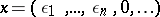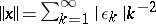# Normed algebra

Jump to: navigation, search

An algebra over the field of real or complex numbers that is at the same time a normed space in which multiplication satisfies some continuity condition. The simplest such condition is separate continuity. Generally speaking, this is weaker than joint continuity in the factors. For example, if one defines the algebraic operations on the set of all finite sequencescoordinate-wise and the norm by, then there arises an algebra in which the multiplication is separately, but not jointly, continuous. The joint continuity of the multiplication in a normed algebra is equivalent to the existence of a constantsuch that. In this and only in this case the completion has the structure of a normed algebra that extends the original one and is a Banach algebra.